## Question of the Week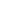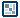Isometric
Transformations
by Danko Kozar : 18 November 2004

Since there aren't many good tutorials online for creating isometric games, I encountered many problems while making my isometric game. Since I am a nice guy, I'd like to share some tips and tricks that I learned, and I hope that they will be inspiring to other Flash developers such as yourself.

These tutorials are all about isometry, or, more specifically, about creating a "filmation" isometric game similar to Knight Lore.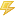Note
 These tutorials can be used for programming an isometric game in many programming languages not just in Flash. They are more about game programming than about Flash programming.

Some of the tutorials that I hope to write in the future will expand upon what I cover today with isometric drawing, hit tests, push-flags, walls and doors handling, maps, depth sorting (which I found to be the hardest part) and all kinds of related stuff.

But, we are getting a little ahead of ourselves. Let's learn about isometric transformations first!

Static isometry
I have to point out that Senocular's tutorials are excellent for the introduction to isometry and it's problems, but there are many things that cannot be done by using this (as I call it) "static isometry" approach.

With "static isometry" I assume that the majority of the objects are "fixed" to the floor, meaning they cannot be moved. Their levels cannot be changed - depth sorting is done by changing the level of the main character only.

Dynamic isometry
Dynamic isometry assumes the following rules:

1. Objects are not fixed to the floor, thus their positions cannot be contained in an array.
2. All of the objects in the room can be pushed and moved.
3. Hit tests are done by comparing every object's position with all other objects through the loop.
4. Depth sorting of objects has to be made dynamically - the level of every object has to be changeable.

The Facts
I'd like to start out with two facts which seems are not so obvious to everyone.Fact Number 1
 All things (calculations etc.) have to be done in 3d coordinate system, and then transformed into 2d using isometric transformations.

I've seen some attempts of trying to use Flash's hitTest function to detect collisions in isometry. But, as I said - they are only attempts, because of rule number 2:Fact Number 2
 Collision detection in isometric projection cannot be done using hitTest function.

The reason you can't use collision detection with hitTest, is that the hitTest function is capable only of calculating 2d, rather than 3d collisions. So we'll have to come up with some other method.

But first, let's take a look at the coordinate systems we will be using.

Coordinate systems and transformations

We have 3 coordinate systems involved in isometric transformation equations: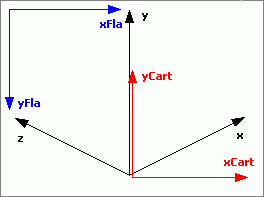[ isometric, cartesian and flash coordinate system ]

An isometric coordinate system in the above image is colored black. The red one is cartesian, and a blue one is Flash coordinate system (which has an offset and reversed Y axis).

The functions for transforming 3d into 2d are:

// transforms x,y,z coordinates into Flash x coordinate
xFla = function (x, y, z) {
// cartesian coordinates
xCart = (x-z)*Math.cos(0.46365);
// flash coordinates
xI = xCart+xOrigin;
return (xI);
};

// transforms x,y,z coordinates into Flash y coordinate
yFla = function (x, y, z) {
// cartesian coordinates
yCart = y+(x+z)*Math.sin(0.46365);
// flash coordinates
yI = -yCart+yOrigin;
return (yI);
};

A few words about these functions:

0.46365 (radians) - it's a “classic” 1:2 isometric angle which lays up perfectly into pixel grid of the computer screen.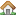Note
 1:2 means that you draw a line moving two pixels right and one pixel up. It slightly differs from a 30º isometric coordinate system, because it is an arctan(1/2), which is actually a 26.57º angle.

xOrigin, yOrigin – offset of isometric and cartesian origins in a Flash coordinate system.

x, y, z – isometric coordinates (black axis in the figure above)
xCart, yCart – cartesian coordinates
xFla, yFla – Flash 2d coordinatesNote
 It is possible to translate coordinates from 3d to 2d only. The other direction doesn’t work because there is a third parameter missing!

Onwards to the next page!